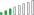cancel
Showing results for
Did you mean:Frequent Visitor

## How do I calculate an extra colomn of Percentage of Grand total with dax

Hello guys,

In the third colomn you can see the  % of GT, this is done by "show value as".

I want this in a measure so I could calculate some formulas.

The column "Total90%FilterCalculate" is created with this measure:

Total90%FilterCalculate = CALCULATE(DISTINCTCOUNT(MKA[Versies.Id]), FILTER(MKA, MKA[VullingTotaalPerc]="90 %"))

How do I create this measure?

Thnx!1 ACCEPTED SOLUTIONCommunity Champion

Hi

try like:
Pct =
VAR _Total=
CALCULATE(
DISTINCTCOUNT(MKA[Versies.Id]),
ALL(MKA)
)
RETURN
FORMAT(DIVIDE([Total90%FilterCalculate], _Total), "0.0%")

@kldefens

3 REPLIES 3Community Champion

Hi

try like:
Pct =
VAR _Total=
CALCULATE(
DISTINCTCOUNT(MKA[Versies.Id]),
ALL(MKA)
)
RETURN
FORMAT(DIVIDE([Total90%FilterCalculate], _Total), "0.0%")

@kldefensResolver IIIFrequent Visitor

I've tried to do the same thing but I get an error. I don't know how to use the measure whenever I use distinccount + filter.